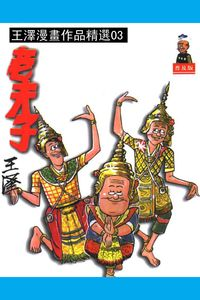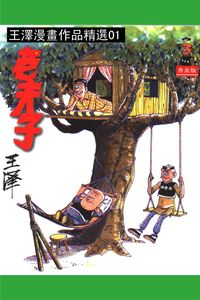### 老夫子. 第2部

• 點閱：19
• 其他題名：漫畫老夫子精選集
• 作者：
• 出版年：1998[民87]
• 出版社：王澤
• 出版地：臺北市
• 集叢名：王澤漫畫作品精選
• 附註：著者本名王家禧

##簡介

《王澤漫畫作品精選：老夫子》風靡港、澳、台及東南亞地區，四十餘年經久不衰，至今總銷量已達六千萬冊以上，堪稱華人漫畫中的經典。

“老夫子”漫畫人物創於1962年。1964年，首期“老夫子”漫畫單行本出版於香港。60年代至70年代，“老夫子”漫畫連載於香港多類報章與雜誌。

《王澤漫畫作品精選：老夫子》並非 ​​完全走寫實諷刺路線。王澤的天馬行空、時空轉移、瘋狂搞笑，更能夠抓緊讀者。有點好色的老父子，可愛憨厚的大番薯，英俊瀟灑的秦先生，三個小人物紮根香港大社會，通過幽默的言行舉止凸現世相萬千，這就是華人傳統漫畫經典《老夫子》中人人樂道的三位主角。

• 意料之外(p.3)
• 來遲一步(p.10)
• 寧死不屈(p.17)
• 照舊(p.24)
• 快馬加鞭(p.31)
• 吸煙禍患(p.38)
• 無地自容(p.45)
• 精神勝利(p.52)
• 補返數(p.59)
• 冷熱相吸(p.66)
• 女流的教訓(p.73)
• 過重(p.80)
• 有口難言(p.87)
• 緣木求魚(p.94)
• 特種道友(p.101)
• 目迷五色(p.108)
• 希特勒二世(p.115)
• 珍禽異獸(p.122)
• 一時大意(p.129)
• 冤家對頭(p.136)
• 疲勞過度(p.143)
• 力不從心(p.150)
• 一無所獲(p.157)
• 逼不得已(p.164)
• 有驚無險(p.171)
• 以卵擊石(p.178)
• 偷雞不成(p.185)
• 奸商的花樣(p.192)
• 烏龍踢球(p.198)
• 有車階級(p.205)
• 無獨有偶(p.212)
• 土包子(p.219)
• 雞鳴狗盜(p.226)
• 一毛之能(p.233)
• 旦夕禍福(p.240)
• 狂人論秋(p.247)
• 不學無術(p.254)
• 絕種貨(p.261)
• 新舊不合(p.268)
• 一去不回(p.275)
• 迷信與野心(p.282)
• 新型紙紮(p.289)
• 風流鬼(p.295)
• 隨機應變(p.302)
• 絕代技術(p.309)
• 不富則貴(p.316)
• 中秋月餅(p.323)
• 胡不歸(p.330)
• 化敵為友(p.337)
• 神腳(p.344)
• 就地取材(p.351)
• 弄巧反拙(p.358)
• 衛生面包(p.365)
• 當眾出醜(p.372)
• 混水摸魚(p.379)
• 水落石出(p.386)
• 加料國畫(p.393)
• 延頸舉踵(p.400)
• 做慈善(p.407)
• 千斤之體(p.414)
• 千金小姐(p.421)
• 弄假成真(p.428)
• 買花豔遇(p.435)
• 神學與科學(p.442)
• 破釜沉舟(p.449)
• 強詞奪理(p.456)
• 陰魂不散(p.463)
• 吹風槍(p.470)
• 的確美麗(p.477)
• 運動家(p.484)
• 杳無信息(p.491)
• 搶風頭(p.498)
• 貌美(p.505)
• 旅行車(p.512)
• 誤會(p.519)
• 得而複失(p.526)
• 釣二手魚(p.533)
• 女人禍水(p.540)
• 面粉頭(p.547)
• 花開花落(p.554)
• 壯志淩雲(p.561)
• 疊羅漢(p.568)
• 追求時髦(p.574)
• 得意忘形(p.581)
• 好人難做(p.588)
• 團團轉(p.595)
• 團團轉(p.602)
• 偏心(p.609)
• 忙中作樂(p.616)
• 人小鬼大(p.623)
• 舍己為人(p.629)
• 特別演員(p.636)
• 回天乏術(p.643)
• 還我河山(p.650)
• 何處不相逢(p.657)
• 不倒翁(p.664)
• 借西裝(p.671)
• 見機而行(p.678)
• 最新秋裝(p.685)
• 十萬火急(p.692)
• 早有預料(p.699)
• 改頭換面(p.706)
• 裝聾作啞(p.713)
• 亡命之徒(p.720)
• 窮達有命(p.727)
• 時運不通(p.734)
• 導電物(p.741)
• 人面獸心(p.748)
• 紅樓琴斷(p.755)
• 新潮舞蹈(p.762)
• 食不果腹(p.769)
• 手足情深(p.776)
• 一身是膽(p.783)
• 入錯籃(p.790)
• 大張旗鼓(p.797)
• 有來有往(p.802)
• 最終一招(p.809)
• 神出鬼沒(p.816)
• 聰明笨伯(p.823)
• 新春打劫(p.830)
• 得不償失(p.837)
• 花開富貴(p.844)
• 新春大雞(p.851)
• 大雞拜年(p.858)
• 天官賜福(p.865)
• 長輩利是(p.872)
• 有來有往(p.879)
• 老羞成怒(p.886)
• 即時趕到(p.893)
• 弄巧反拙(p.900)
• 單槍赴會(p.907)
• 長氣(p.914)
• 四海為家(p.921)
• 自作聰明(p.928)
• 放生(p.935)
• 自作自受(p.942)
• 美中不足(p.949)
• 小題大做(p.956)
• 不分皂白(p.963)
• 少管閑事(p.970)
• 新式娛樂(p.977)
• 操之過急(p.984)
• 愛美之過(p.991)
• 好辦法(p.998)
• 大小唱片(p.1004)
• 視死如歸(p.1011)
• 同門異戶(p.1018)
• 雙份工作(p.1025)
• 席卷而去(p.1032)
• 舒服過頭(p.1039)
• 卷土重來(p.1046)
• 臨陣脫逃(p.1053)
• 特別藝術(p.1060)
• 英雄末路(p.1067)
• 虎口餘生(p.1074)
• 先恐後爭(p.1081)
• 落井下石(p.1088)
• 假發(p.1095)
• 過度緊張(p.1102)
• 假日交通(p.1109)
• 智退盜賊(p.1116)
• 血肉橫飛(p.1123)
• 莫名奇妙(p.1130)
• 萬能遊艇(p.1137)
• 發酒瘋(p.1144)

##同作者書籍老夫子. 第3部老夫子. 第1部